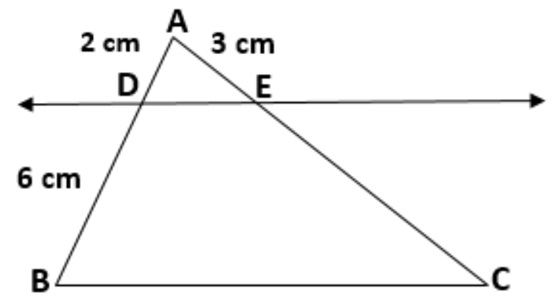CBSE Class 10 Sample Paper for 2023 Boards - Maths Basic

Class 10
Solutions of Sample Papers for Class 10 Boards

## Prove that If a line is drawn parallel to one side of a triangle to intersect the other two sides in distinct points, the other two sides are divided in the same ratio. In the figure, find EC if AB/DB=AE/EC using the above theoremThis question is Similar to Theorem 6.1 - Basic Proportionality Theorem - Chapter 6 Class 10

Learn in your speed, with individual attention - Teachoo Maths 1-on-1 Class